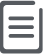# 算法原理-快速排序2021-02-07JavaScript1168

1. 在数据集之中，选择一个元素作为”基准”（pivot）。

2. 所有小于”基准”的元素，都移到”基准”的左边；所有大于”基准”的元素，都移到”基准”的右边。这个操作称为分区 (partition) 操作，分区操作结束后，基准元素所处的位置就是最终排序后它的位置。

3. 对”基准”左边和右边的两个子集，不断重复第一步和第二步，直到所有子集只剩下一个元素为止。
分区是快速排序的主要内容，用伪代码可以表示如下

``````function partition(a, left, right, pivotIndex)
pivotValue := a[pivotIndex]
swap(a[pivotIndex], a[right]) // 把 pivot 移到結尾
storeIndex := left
for i from left to right-1
if a[i] < pivotValue
swap(a[storeIndex], a[i])
storeIndex := storeIndex + 1
swap(a[right], a[storeIndex]) // 把 pivot 移到它最後的地方
return storeIndex // 返回 pivot 的最终位置``````

``````procedure quicksort(a, left, right)
if right > left
select a pivot value a[pivotIndex]
pivotNewIndex := partition(a, left, right, pivotIndex)
quicksort(a, left, pivotNewIndex-1)
quicksort(a, pivotNewIndex+1, right)``````

## 实例分析

1. 首先选定一个基准元素，这里我们元素 5 为基准元素（基准元素可以任意选择）：
``````          pivot
↓
3   7   8   5   2   1   9   5   4``````
1. 将基准元素与数组中最后一个元素交换位置，如果选择最后一个元素为基准元素可以省略该步：
``````                              pivot
↓
3   7   8   4   2   1   9   5   5``````
1. 从左到右（除了最后的基准元素），循环移动小于基准元素 5 的所有元素到数组开头，留下大于等于基准元素的元素接在后面。在这个过程它也为基准元素找寻最后摆放的位置。循环流程如下

``````                                    pivot
↓
3   7   8   4   2   1   9   5   5
↑
storeIndex``````

``````         ┌───────┐                 pivot
↓       ↓                   ↓
3   7   8   4   2   1   9   5   5
↑       ↑
storeIndex   i``````
``交换位置后，storeIndex 自增 1，storeIndex == 2：``
``````                                  pivot
↓
3   4   8   7   2   1   9   5   5
↑
storeIndex``````
``循环 i == 4 时，storeIndex == 2，找到一个小于基准元素的元素 2：``
``````            ┌───────┐             pivot
↓       ↓               ↓
3   4   8   7   2   1   9   5   5
↑       ↑
storeIndex   i``````
``交换位置后，storeIndex 自增 1，storeIndex == 3：``
``````                                  pivot
↓
3   4   2   7   8   1   9   5   5
↑
storeIndex``````
``````循环 i == 5 时，storeIndex == 3，找到一个小于基准元素的元素 1：

```
┌───────┐         pivot
↓       ↓           ↓
3   4   2   7   8   1   9   5   5
↑       ↑
storeIndex   i
```

``````                                  pivot
↓
3   4   2   1   8   7   9   5   5
↑
storeIndex``````
``循环 i == 7 时，storeIndex == 4，找到一个小于等于基准元素的元素 5：``
``````                    ┌───────────┐ pivot
↓           ↓   ↓
3   4   2   1   8   7   9   5   5
↑           ↑
storeIndex       i``````
``交换后位置后，storeIndex 自增 1，storeIndex == 5：``
``````                                  pivot
↓
3   4   2   1   5   7   9   8   5
↑
storeIndex``````
1. 循环结束后交换基准元素和 storeIndex 位置的元素的位置：
``````                  pivot
↓
3   4   2   1   5   5   9   8   7
↑
storeIndex``````

## JavaScript 语言实现

``````function quickSort(arr) {
if (arr.length <= 1) {
return arr;
}
var pivotIndex = Math.floor(arr.length / 2);
var pivot = arr.splice(pivotIndex, 1);
var left = [];
var right = [];
for (var i = 0; i < arr.length; i++) {
if (arr[i] < pivot) {
left.push(arr[i]);
} else {
right.push(arr[i]);
}
}
return quickSort(left).concat([pivot], quickSort(right));
}``````

``````function quickSort(array) {
// 交换元素位置
function swap(array, i, k) {
var temp = array[i];
array[i] = array[k];
array[k] = temp;
}
// 数组分区，左小右大
function partition(array, left, right) {
var storeIndex = left;
var pivot = array[right]; // 直接选最右边的元素为基准元素
for (var i = left; i < right; i++) {
if (array[i] < pivot) {
swap(array, storeIndex, i);
storeIndex++; // 交换位置后，storeIndex 自增 1，代表下一个可能要交换的位置
}
}
swap(array, right, storeIndex); // 将基准元素放置到最后的正确位置上
return storeIndex;
}
function sort(array, left, right) {
if (left > right) {
return;
}
var storeIndex = partition(array, left, right);
sort(array, left, storeIndex - 1);
sort(array, storeIndex + 1, right);
}
sort(array, 0, array.length - 1);
return array;
}``````

``````function quickSort(arr) {
return sort(arr, 0, arr.length - 1);
function swap(arr, i, k) {
var temp = arr[i];
arr[i] = arr[k];
arr[k] = temp;
}
function sort(arr, start, end) {
sort(arr, 0, arr.length - 1);
return arr;
function swap(arr, i, k) {
var temp = arr[i];
arr[i] = arr[k];
arr[k] = temp;
}
function sort(arr, start, end) {
if (start >= end) return;
var pivot = arr[start],
i = start + 1,
k = end;
while (true) {
while (arr[i] < pivot) {
i++;
}
while (arr[k] > pivot) {
k--;
}
if (i >= k) {
break;
}
swap(arr, i, k);
}
swap(arr, start, k);
sort(arr, start, Math.max(0, k - 1));
sort(arr, Math.min(end, k + 1), end);
}
}
}``````

## 参考文章

PREVIOUS:
NEXT: